• Submissions# Control of a SCARA Robot Using Feedback Linearization and Kalman Filter Observer

Saeid Reza Nazari*, Mojtaba Vakilian and Zahra Kheirandish

Department of Mechanical Engineering, Iran

*Corresponding author:Saeid Reza Nazari, Department of Mechanical Engineering, Iran

Submission: June 21, 2018;Published: February 21, 2019

DOI: 10.31031/EME.2019.02.000539ISSN 2640-9690
Volume2 Issue3

#### Abstract

In this paper an efficient controller is presented for controlling SCARA robot in present of undesirable noises in variable states. To do so, feedback linearization is considered as the main controller and kalman filter estimator is utilized to estimate the state variables from the noisy output signals. In order to evaluate the performance of the purposed method, a simulation test is performed to apply this controller on the SCARA robot. The simulation is done for both noisy and not noisy signals. The results show that the kalman filter observer has accomplished a good state estimation and feedback lineation controller has tracked the desired signals perfectly as well.

Keywords: SCARA robot stochastic; Kalman filter; LQE; Disturbance

#### Introduction

The importance of using robot in daily life is shown, when human life is at risk. For instance, in high-risk industrial places such as working alongside the melting furnaces or neutralize mines on the battlefield and such high-risk areas. In additional, human accuracy is reduced when doing repetitive work. Mentioned reasons, the use of robots has become prevalent in many diverse industries and locations [1-8]. SCARA robot is a kind of usual robots which is used for welding, painting, assembly and material handling . This robot consists of three degree of freedom. Economic justification for the cost of installing a robot must be considered some important things. The robot control system must have some necessities like short time of cycling, continuous and accurately tracking. To achieve the short time cycling; the robot needs to have enough fast response, to get to high tracking accuracy, the tracking error should be very low . The importance problem in using of the robot is accurate control method to guiding the end effector to the desired position quickly.

There are various applicable controller methods that can be divided in to different branches such as model-base or none modelbase [11-21]; (Figure 1 & 2), adaptive [22-28], and intelligence [29- 36] methods. Tuning the parameters of a controller method which contains a high number of tunable parameters, is very crucial and can be very cumbersome. To accomplish this process, one way which is very common, is employing an efficient optimization method [37-95]. By determining a proper cost function, the optimization algorithm can tune the controller parameters optimally. It worth nothing that, selecting a power full optimization method is very vital and must be done regarding the number of tunable parameters and the complexity of the ultimate cost function. Recently, some novel and effective population optimization algorithms have been presented that can be used for this goal.

The aim of this paper is to introduce an applicable and effective controller for controlling a SCARA robot in present of disturbance. To do so, feedback linearization (FL) method which is a nonlinear and effective controller is selected. Sensitivity to noise is a disadvantage of FL. To overcome this problem using a kalman filter to estimate the state variables is suggested.

This paper complained of several sections. Section 2 described problems, parameter and explained the assumptions. In section 3 is reported the dynamic modeling direct kinematic of SCARA robot. 4th section depicted the controller. Stochastic feedback linearization LQG with Kalman filter is used to control the SCARA manipulator robot. This controller can estimate parameter and identified original signal in comparison of disturbance signals. In section 5 demonstrated the results simulation by Figure 1 and curves. Figure 1 of tracking is pictured in this section also, which validated the successful performance of controller. Finally, in ending section explained the conclusions of paper.

#### Problem Description

In this paper the SCARA robot with 3 degree of freedom is controlled. This robot is RRP type and is assumed all of arms is rigid body. The friction in each joint is negligible. The original mass moment of inertia is in Z-direction. There is no mass moment of inertia in other plane. The final joint has a force in Z-direction which is shown in Figure 1. G1 and G2 are manipulator center of mass.

#### The Governing SCARA Dynamical Equations

In this section the dynamic equation of SCARA robot is obtained. For this purpose, the Euler-Lagrangian method is used to derive dynamic equations. So, the kinematic and potential energy of the system must be computed to use in Lagrangian equation. The kinematic and potential energy of the system is obtained as :Where xci and yci are the center of mass position of ith manipulator, m1,m2 and m3 are mass of manipulators, Ic1 and Ic2are the moment of inertia around center of mass manipulator 1 and 2 respectively and i è is the angel of the th i manipulator which is shown in Figure 2, g is the gravity acceleration and z is the height of center of mass manipulator 3. xci and yci are :So, the velocity of each manipulator isWhere Lci and Lci are the distance between center of mass ith manipulator and ith joint.

So, the Lagrangian of the system is obtained as [4,11,34]:

L = K −U

Where L is the Lagrangian function. By using the Euler-Lagrange formulation the dynamic equation of the system is obtained as below: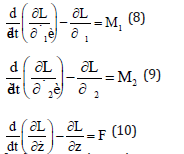In these equations q is states of the system and defined as: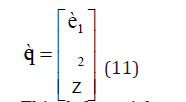This deferential equation can be expressed in matrix form as:Where the matrices M, C, and G are represented the 3×3 inertia matrix, 3×1 Centripetal-Coriolis

Matrix terms, and 3×1gravity matrix, respectively and T are 3×1 matrix which is defined as below:In state space the state is defined as below:#### Controller Design

In this section the stochastic LQE feedback linearization controller with Kalman filter, is designed. Feedback linearization: In feedback linearization method by equaling the nonlinear system in to a stable linear system, the control law can be derived as nonlinear system as below [1,16,26]. The stable linear system has been considered as below:Where matrix A and B be are defied as: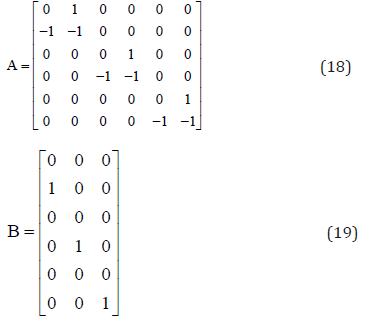Where E is the vector of state variable of the linear system and V is system input vector. The nonlinear system can be obtained also as:Where X is the state variable of the nonlinear system, F and G are derived from equations 14 to 16.

With considering E as error we have:So, the controller law for nonlinear system derived as:### LQE controller

In control theory, the Linear-Quadratic-Estimator (LQE) control problem is one of the most fundamental optimal control problems . It concerns uncertain linear systems disturbed by additive white Gaussian noise, having incomplete state information and undergoing control subject to quadratic costs. Moreover, the solution is unique and constitutes a linear dynamic feedback control law that is easily computed and implemented (Figure 3). Finally, the LQE controller is also fundamental to the optimal control of perturbed non-linear systems.

The linear system is considered as below:In this problem C is 6x6 identity matrix.

Where the vector E is state variable, the vector V is control input, the vector Y is measurement output and the vector n are additive white Gaussian system noise and w is additive white Gaussian measurement noise. The LQE controller is specified by the following equations:Where the matrix K is Kalman gain which is obtained from Kalman filter equation.WhereWhere in the above differential equations W the covariance of w and N is the covariance of n.

#### Simulation Result

To regulate the angle system by controller 1, 2 θ θ and z are main parameters which are considered. The simulation results are Investigation in two Conditions, one with present disturbance and the other without consider disturbance. Also, the Measured and Estimated states are illustrated in Figure 4a-4f for regulation and in Figure 5a-5f for tracking purpose.

#### Conclusion

In this investigation, the SCARA robot control is presented. SCARA robot is controlled by a stochastic feedback linearization LQE with Kalman filter. This controller can control the robot successfully and identified original signals in presence of the disturbance’s signals. In feedback linearization method by equaling the nonlinear system in to a stable linear system, the control law can be derived as nonlinear system. LQE controller concerns uncertain linear systems disturbed by additive white Gaussian noise, having incomplete state information and undergoing control subject to quadratic costs. This combination of useful control methods is already set to lead the system to the desired position in presence of disturbance as is evidenced in the simulation results and Figure 1-5.

Figure 1:Schematic of front view of SCARA robot .Figure 2:Schematic of left view of SCARA robot .Figure 3:Schematic Stochastic controller.Figure 4:Estimation of the state variables, a) θ1without noise, b) θ1with noise, c) θ2 without noise, d) θ2 with noise, e) z without noise, f) z with noise.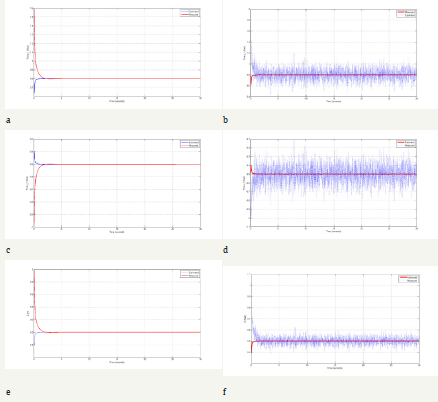Figure 5:Regulation for variable state, a) θ1 without noise, b) θ1 with noise, c) θ2 without noise ,d) θ2 with noise, e) z without noise ,f) z with noise.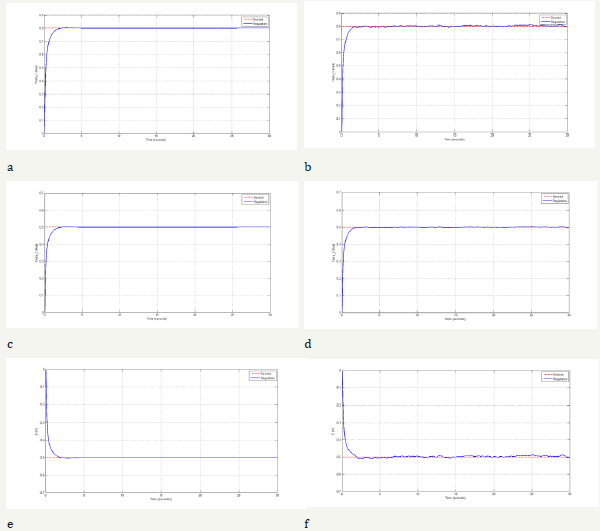We at Crimson Publishing are a group of people with a combined passion for science and research, who wants to bring to the world a unified platform where all scientific know-how is available read more...